# Simulate functions of the package

#### 2020-06-16

Currently “smooth” function contains simulation function for Exponential Smoothing (es) and SARIMA (ssarima). It is planned to produce other functions for other state-space models. This is part of smooth package.

require(smooth)

## Exponential Smoothing

We start with an Exponential Smoothing. For example, monthly data generated from ETS(A,N,N), 120 observations:

ourSimulation <- sim.es("ANN", frequency=12, obs=120)

The resulting ourSimulation object contains: ourSimulation$model – name of ETS model used in simulation; ourSimulation$data – vector of simulated data; ourSimulation$states – matrix of states, where columns contain different states and rows corresponds to time; ourSimulation$persistence – vector of smoothing parameters used in simulation (in our case generated randomly); ourSimulation$residuals – vector of errors generated in the simulation; ourSimulation$occurrence – vector of demand occurrences (zeroes and ones, in our case only ones); ourSimulation$logLik – true likelihood function for the used generating model. We can plot produced data, states or residuals in order to see what was generated. This is done using: plot(ourSimulation$data)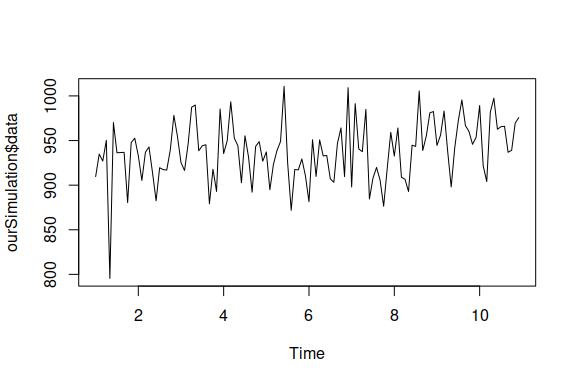If only one time series has been generated, we can use a simpler command in order to plot it:

plot(ourSimulation)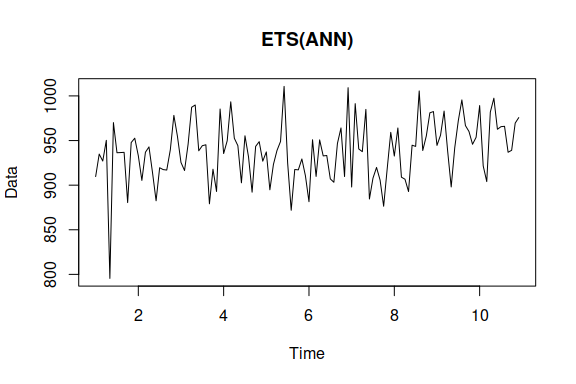Now let’s use more complicated model and be more specific, providing persistence vector:

ourSimulation <- sim.es("MAdM", frequency=12, obs=120, phi=0.95, persistence=c(0.1,0.05,0.01))
plot(ourSimulation)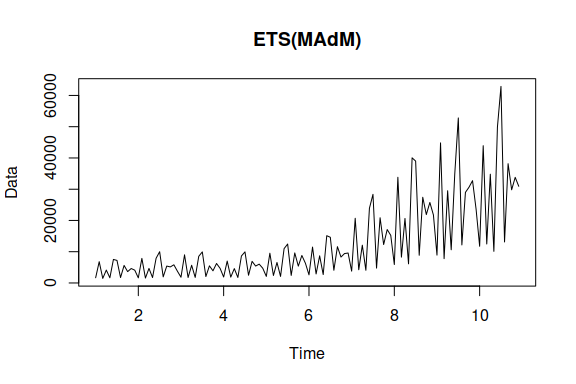High values of smoothing parameters are not advised for models with multiplicative components, because they may lead to explosive data. As for randomizer the default values seem to work fine in the majority of cases, but if we want, we can intervene and ask for something specific (for example, some values taken from some estimated model):

ourSimulation <- sim.es("MAdM", frequency=12, obs=120, phi=0.95, persistence=c(0.1,0.05,0.01), randomizer="rlnorm", meanlog=0, sdlog=0.015)
plot(ourSimulation)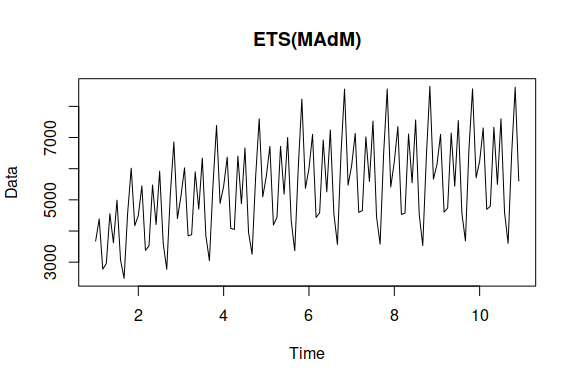It is advised to use lower values for sdlog and sd for models with multiplicative components. Once again, using higher values may lead to data with explosive behaviour.

If we need intermittent data, we can define probability of occurrences. And it also makes sense to use pure multiplicative models and specify initials in this case:

ourSimulation <- sim.es("MNN", frequency=12, obs=120, probability=0.2, initial=10, persistence=0.1)
plot(ourSimulation)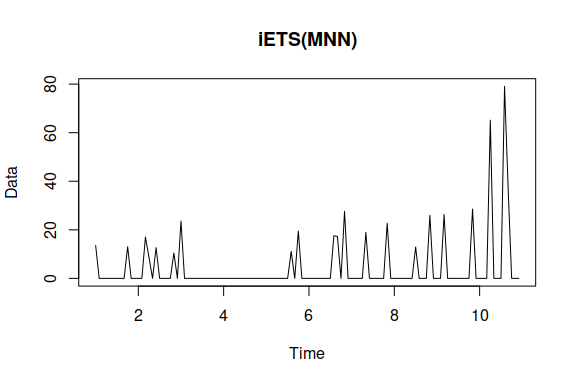If we want to have several time series generated using the same parameters then we can use nsim parameter:

ourSimulation <- sim.es("MNN", frequency=12, obs=120, probability=0.2, initial=10, persistence=0.1, nsim=50)

We will have the same set of returned values, but with one more dimension. So, for example, we will end up with matrix for ourSimulation$data and array for ourSimulation$states.

There is also simulate() function that allows to simulate data from estimated ETS model. For example:

x <- ts(rnorm(100,120,15),frequency=12)
ourModel <- es(x, h=18, silent=TRUE)
ourData <- simulate(ourModel, nsim=50, obs=100)

Now let’s compare the original data and the simulated one:

par(mfcol=c(1,2))
plot(x)
plot(ourData$data[,1]) par(mfcol=c(1,1))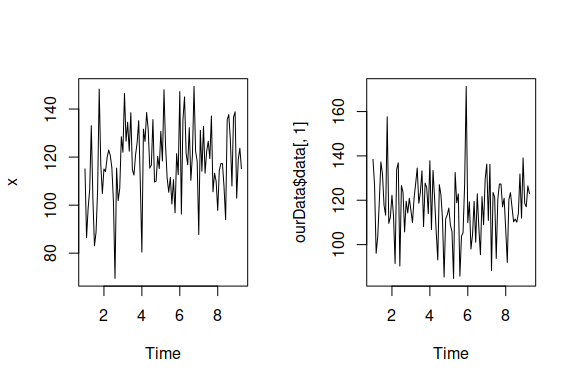As we see the level is the same and variance is similar for both series. Achievement unlocked! ## SARIMA Let’s start from something simple. By default sim.ssarima() generates data from ARIMA(0,1,1). Let’s produce 10 time series with 120 observations of that: ourSimulation <- sim.ssarima(frequency=12, obs=120, nsim=10) The resulting ourSimulation object contains: ourSimulation$model – name of ARIMA model used in simulation; ourSimulation$AR – matrix with generated or provided AR parameters, ourSimulation$MA – matrix with MA parameters, ourSimulation$AR – vector with constant values (one for each time series), ourSimulation$initial – matrix with initial values, ourSimulation$data – matrix of simulated data (if we had nsim=1, then that would be a vector); ourSimulation$states – array of states, where columns contain different states, rows corresponds to time and last dimension is for each time series; ourSimulation$residuals – vector of errors generated in the simulation; ourSimulation$occurrence – vector of demand occurrences (zeroes and ones, in our case only ones); ourSimulation$logLik – true likelihood function for the used generating model. Similarly to sim.es(), we can plot produced data, states or residuals for each time series in order to see what was generated. Here’s an example: plot(ourSimulation$data[,5])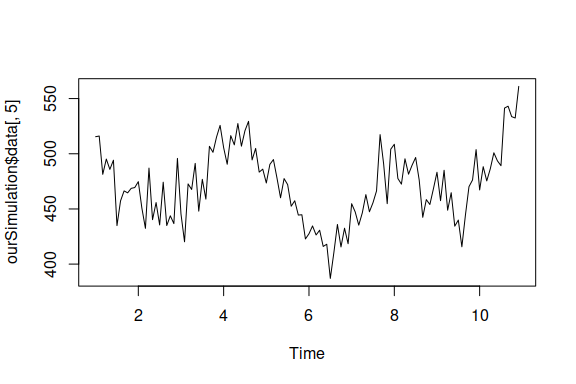Now let’s use more complicated model. For example, data from SARIMA(0,1,1)(1,0,2)_12 with drift can be generated using:

ourSimulation <- sim.ssarima(orders=list(ar=c(0,1),i=c(1,0),ma=c(1,2)), lags=c(1,12), constant=TRUE, obs=120)
plot(ourSimulation)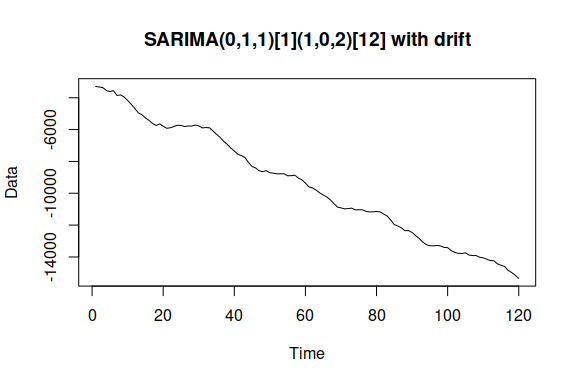If we want to provide some specific parameters, then we should follow the structure: from lower lag to lag from lower order to higher order. For example, the same model with predefined MA terms will be:

ourSimulation <- sim.ssarima(orders=list(ar=c(0,1),i=c(1,0),ma=c(1,2)), lags=c(1,12), constant=TRUE, MA=c(0.5,0.2,0.3), obs=120)
ourSimulation
## Data generated from: SARIMA(0,1,1)(1,0,2) with drift
## Number of generated series: 1
## AR parameters:
##       Lag 12
## AR(1) 0.7683
## MA parameters:
##       Lag 1 Lag 12
## MA(1)   0.5    0.2
## MA(2)   0.0    0.3
## Constant value: -88.5825
## True likelihood: -728.0355

We can create time series with several frequencies For example, some sort of daily series from SARIMA(1,0,2)(0,1,1)_7(1,0,1)_30 can be generated with a command:

ourSimulation <- sim.ssarima(orders=list(ar=c(1,0,1),i=c(0,1,0),ma=c(2,1,1)), lags=c(1,7,30), obs=360)
ourSimulation
## Data generated from: SARIMA(1,0,2)(0,1,1)(1,0,1)
## Number of generated series: 1
## AR parameters:
##        Lag 1 Lag 30
## AR(1) 0.4652 0.7549
## MA parameters:
##        Lag 1   Lag 7 Lag 30
## MA(1) 0.5552 -0.4736 0.9603
## MA(2) 0.7970  0.0000 0.0000
## True likelihood: -1758.1804
plot(ourSimulation)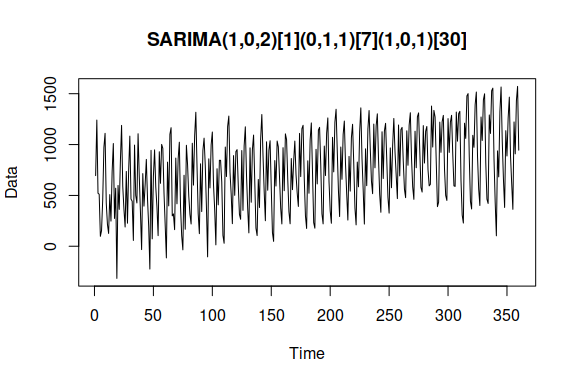sim.ssarima also supports intermittent data, which is defined via probability parameter, similar to sim.es():

ourSimulation <- sim.ssarima(orders=list(ar=c(1,0),i=c(0,1),ma=c(2,1)), lags=c(1,7), obs=120, probability=0.2)
ourSimulation
## Data generated from: iSARIMA(1,0,2)(0,1,1)
## Number of generated series: 1
## AR parameters:
##         Lag 1
## AR(1) -0.2103
## MA parameters:
##        Lag 1  Lag 7
## MA(1) 0.6390 0.2967
## MA(2) 0.4561 0.0000
## True likelihood: -602.8587
plot(ourSimulation)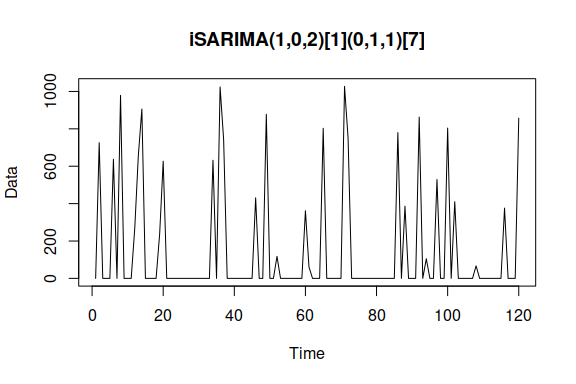Finally we can use simulate() function in a similar manner as with sim.es(). For example:

x <- ts(100 + c(1:100) + rnorm(100,0,15),frequency=12)
ourModel <- auto.ssarima(x, h=18, silent=TRUE)
ourData <- simulate(ourModel, nsim=50, obs=100)

If we don’t want to use everything from the estimated function and need, for example, to use orders only, then we can extract them using orders() and lags() functions:

ourData <- sim.ssarima(orders=orders(ourModel), lags=lags(ourModel), nsim=50, obs=100)

Now let’s compare the original data and one of series from the simulated array:

par(mfcol=c(1,2))
plot(x)
plot(ourData)
par(mfcol=c(1,1))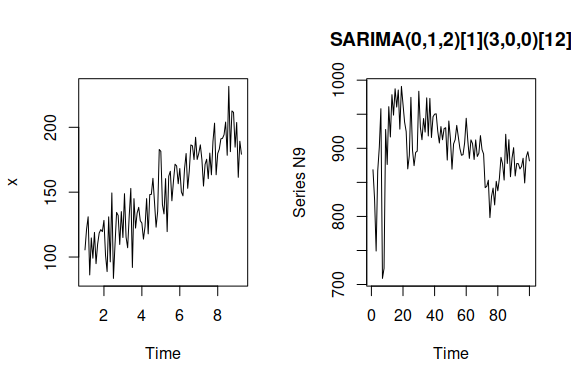As we see series demonstrate similarities in dynamics and have similar variances.

## Complex Exponential Smoothing

As usual we start from a simple model. By default sim.ces() generates data from CES(n). If we do not provide specific parameters, then they will be automatically generated:

ourSimulation <- sim.ces(frequency=12, obs=120, nsim=1)

The resulting ourSimulation object contains: ourSimulation$model – name of CES model used in simulation; ourSimulation$A – vector of complex smoothing parameters A, ourSimulation$B – vector of complex smoothing parameters A (if “partial” or “full” seasonal model was used in the simulation), ourSimulation$initial – array with initial values, ourSimulation$data – matrix of simulated data (if we had nsim=1, then that would be a vector); ourSimulation$states – array of states, where columns contain different states, rows corresponds to time and last dimension is for each time series; ourSimulation$residuals – vector of errors generated in the simulation; ourSimulation$occurrence – vector of demand occurrences (zeroes and ones, in our case only ones); ourSimulation$logLik – true likelihood function for the used generating model. Similarly to other simulate functions in “smooth”, we can plot produced data, states or residuals for each time series in order to see what was generated. Here’s an example: plot(ourSimulation)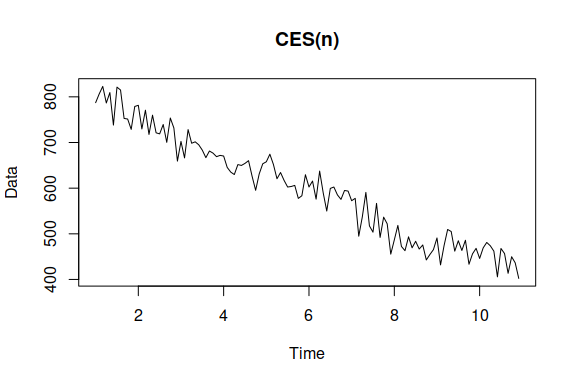We can also see a brief summary of our simulated data: ourSimulation ## Data generated from: CES(n) ## Number of generated series: 1 ## Smoothing parameter a: 1.1537+0.9933i ## True likelihood: -564.2519 We can produce one out of three possible seasonal CES models. For example, Let’s generate data from “Simple CES”, which does not have a level: ourSimulation <- sim.ces("s",frequency=24, obs=240, nsim=1) plot(ourSimulation)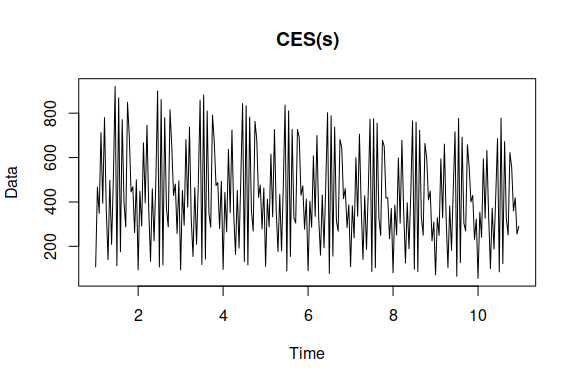Now let’s be more creative and mess around with the generated initial values of the previous model. We will make some of them equal to zero and regenerate the data: ourSimulation$initial[c(1:5,20:24),] <- 0
ourSimulation <- sim.ces("s",frequency=24, obs=120, nsim=1, initial=ourSimulation$initial, randomizer="rt", df=4) plot(ourSimulation)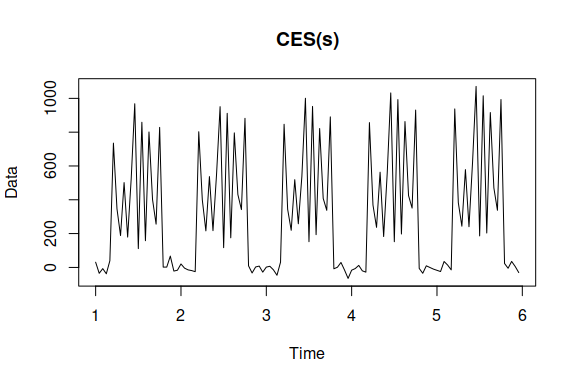The resulting generated series has properties close to the ones that solar irradiation data has: changing amplitude of seasonality without changes in level. We have also chosen a random number generator, based on Student distribution rather than normal. This is done just in order to show what can be done using simulate functions in “smooth”. We can also produce CES with so called “partial” seasonality, which corresponds to CES(n) with additive seasonal components. Let’s produce 10 of such time series: ourSimulation <- sim.ces("p",b=0.2,frequency=12, obs=240, nsim=10) plot(ourSimulation)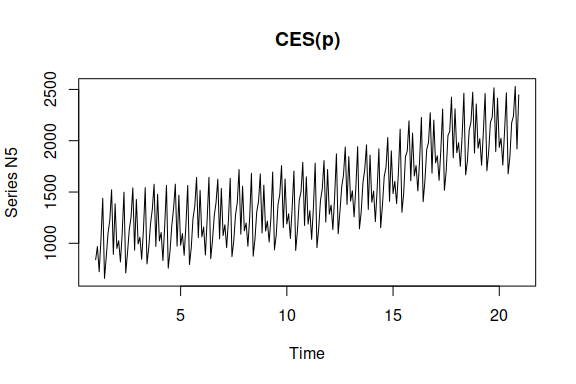Finally, the most complicated CES model is the one with “full” seasonality, implying that there are two complex exponential smoothing models inside: one for seasonal and the other for non-seasonal part: ourSimulation <- sim.ces("f",frequency=12, obs=240, nsim=10) plot(ourSimulation)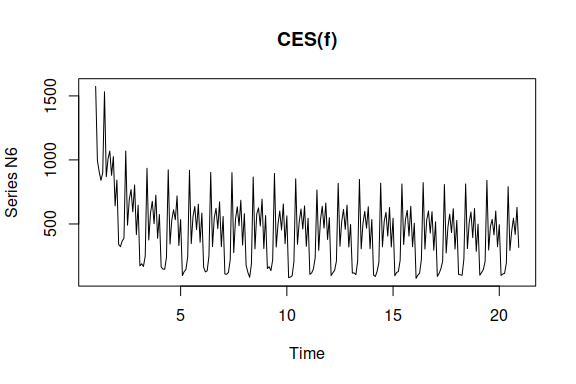The generated smoothing parameters may sometimes lead to explosive behaviour and produce meaningless time series. That is why it is advised to use parameters of a model fitted to time series of interest. For example, here we generate something crazy and then simulate the data: x <- ts(rnorm(120,0,5) + rep(runif(12,-50,50),10)*rep(c(1:10),each=12) ,frequency=12) ourModel <- ces(x, seasonality="s", h=18, silent=TRUE) ourData <- simulate(ourModel, nsim=50, obs=100) Comparing the original data with the simulated one, we will see some similarities: par(mfcol=c(1,2)) plot(x) plot(ourData) par(mfcol=c(1,1))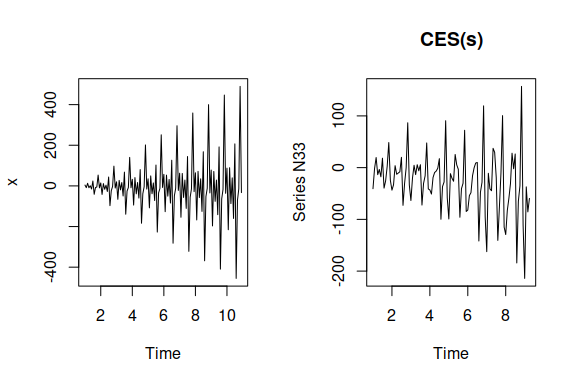## Generalised Exponential Smoothing There is also sim.gum() function in the package that generates data from GUM model. If we do not provide specific parameters, then they will be automatically generated. This model is very flexible and taking that there is a lot of parameters to set, in general it is not advised to leave such parameters as measurement, transition and persistence blank. The good practice is to simulate data from a fitted model: x <- ts(100 + rnorm(120,0,5) + rep(runif(12,-50,50),10)*rep(c(1:10),each=12) ,frequency=12) ourModel <- auto.gum(x, h=18, silent=TRUE) ourData <- simulate(ourModel, nsim=50) Comparing the original data with the simulated one, we will see some similarities: par(mfcol=c(1,2)) plot(x) plot(ourData) par(mfcol=c(1,1))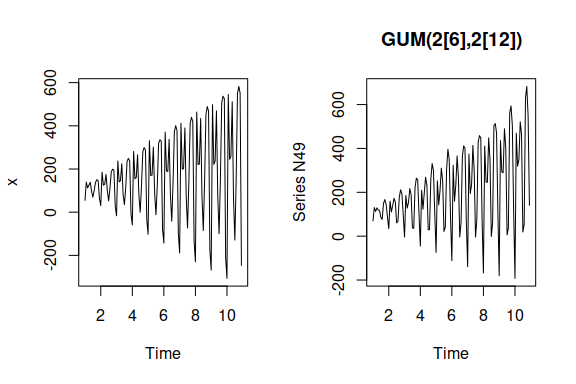Note that GUM is still an ongoing research and its properties are currently understudied. ## Simple Moving Average Now that there is a model underlying simple moving averages, we can simulate the data for it. Here how it can be done: ourSimulation <- sim.sma(10,frequency=12, obs=240, nsim=1) plot(ourSimulation)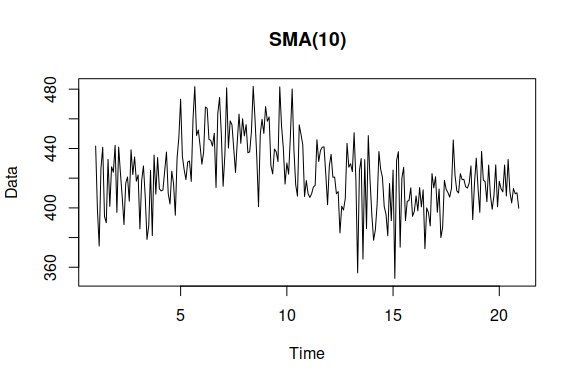As usual, you can use simulate function as well: x <- ts(rnorm(100,100,5), frequency=12) ourModel <- sma(x) ourData <- simulate(ourModel, nsim=50, obs=1000) plot(ourData)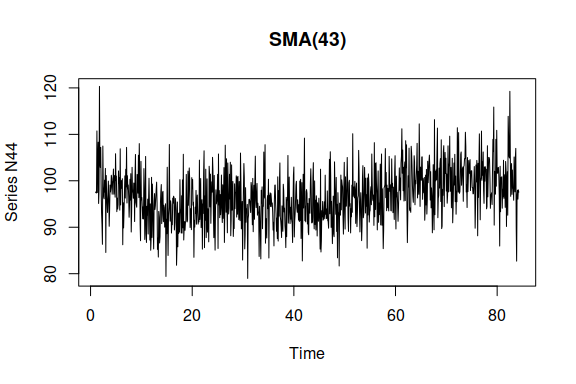## Vector Exponential Smoothing In addition starting from smooth v2.4.5 there is now an option of simulating data from VES model. As an example, we start from the scratch: x <- sim.ves("ANN",frequency=4,obs=40,nSeries=3,randomizer="rnorm",mean=0,sd=100) This way the we generate two independent time series with similar DGPs and the same parameters (initials, persistence etc). We can use options similar to the ones used in sim.es() in order to specify a more flexible model. In addition, we can simulate multivariate series from an estimated VES model: ourModel <- ves(x$data,model="AAN",persistence="dependent")
Y <- simulate(ourModel)
plot(Y)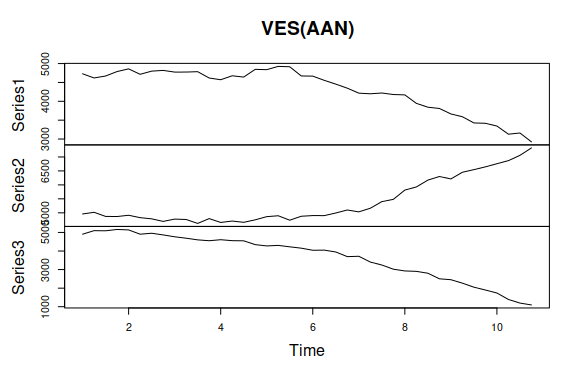When using simulate with ves, you can specify randomizer and additional parameters for distributions. For example, you can use mvrnorm() from MASS package and if you don’t provide mu and Sigma then they will be extracted from the model.